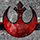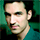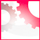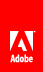Accelerometer input

Flash Player 10.1 and later, Adobe AIR 2 and later

The Accelerometer class dispatches events based on activity detected by the device's motion sensor. This data represents the device's location or movement along a three-dimensional axis. When the device moves, the sensor detects this movement and returns the acceleration coordinates of the device. The Accelerometer class provides methods to query whether accelerometer is supported, and also to set the rate at which acceleration events are dispatched.

The accelerometer axes are normalized to the display orientation, not the physical orientation of the device. When the device re-orients the display, the accelerometer axes are re-oriented as well. Thus the y-axis is always roughly vertical when the user is holding the phone in a normal, upright viewing position — no matter which way the phone is rotated. If auto-orientation is off, for example, when SWF content in a browser is in full-screen mode, then the accelerometer axes are not re-oriented as the device is rotated.Flash Pro CS5.5 Mobile (Android/iOS) – Part 2: Project 2 Accelerometer and DebuggingAIR and the AccelerometerChecking accelerometer support

Use the Accelerometer.isSupported property to test the runtime environment for the ability to use this feature:

if (Accelerometer.isSupported)
{
// Set up Accelerometer event listeners and code.
}

The Accelerometer class and its members are accessible to the runtime versions listed for each API entry. However the current environment at run time determines the availability of this feature. For example, you can compile code using the Accelerometer class properties for Flash Player 10.1, but you need to use the Accelerometer.isSupported property to test for the availability of the Accelerometer feature on the user’s device. If Accelerometer.isSupported is true at runtime, then Accelerometer support currently exists.

Detecting accelerometer changes

To use the accelerometer sensor, instantiate an Accelerometer object and register for update events it dispatches. The update event is an Accelerometer event object. The event has four properties, and each are numbers:

• accelerationX —Acceleration along the x-axis, measured in g’s. The x-axis runs from the left to the right of the device when it is in the upright position. (The device is upright when the top of the device is facing up.) The acceleration is positive if the device moves toward the right.

• accelerationY —Acceleration along the y-axis, measured in g’s. The y-axis runs from the bottom to the top of the device when it is in the upright position. (The device is upright when the top of the device is facing up.) The acceleration is positive if the device moves up relative to this axis.

• accelerationZ —Acceleration along the z-axis, measured in g’s. The Z axis runs perpendicular to the face of the device. The acceleration is positive if you move the device so that the face of the device points up. The acceleration is negative if the face of the device points towards the ground.

• timestamp —The number of milliseconds at the time of the event since the runtime was initialized.

1 g is the standard acceleration due to gravity, roughly 9.8 m/sec 2. .

Here is a basic example that displays accelerometer data in a text field:

var accl:Accelerometer;
if (Accelerometer.isSupported)
{
accl = new Accelerometer();
}
else
{
accTextField.text = "Accelerometer feature not supported";
}
function updateHandler(evt:AccelerometerEvent):void
{
accTextField.text = "acceleration X: " + evt.accelerationX.toString() + "\n"
+ "acceleration Y: " + evt.accelerationY.toString() + "\n"
+ "acceleration Z: " + evt.accelerationZ.toString()
}

To use this example, be sure to create the accTextField text field and add it to the display list before using this code.

You can adjust the desired time interval for accelerometer events by calling the setRequestedUpdateInterval() method of the Accelerometer object. This method takes one parameter, interval , which is the requested update interval in milliseconds:

var accl:Accelerometer;
accl = new Accelerometer();
accl.setRequestedUpdateInterval(1000);

The actual time between accelerometer updates may be greater or lesser than this value. Any change in the update interval affects all registered listeners. If you don’t call the setRequestedUpdateInterval() method, the application receives updates based on the device's default interval.

Accelerometer data has some degree of inaccuracy. You can use a moving average of recent data to smooth out the data. For example, the following example factors recent accelerometer readings with the current reading to get a rounded result:

var accl:Accelerometer;
var rollingX:Number = 0;
var rollingY:Number = 0;
var rollingZ:Number = 0;
const FACTOR:Number = 0.25;

if (Accelerometer.isSupported)
{
accl = new Accelerometer();
accl.setRequestedUpdateInterval(200);
}
else
{
accTextField.text = "Accelerometer feature not supported";
}
function updateHandler(event:AccelerometerEvent):void
{
accelRollingAvg(event);
accTextField.text = rollingX + "\n" +  rollingY + "\n" + rollingZ + "\n";
}

function accelRollingAvg(event:AccelerometerEvent):void
{
rollingX = (event.accelerationX * FACTOR) + (rollingX * (1 - FACTOR));
rollingY = (event.accelerationY * FACTOR) + (rollingY * (1 - FACTOR));
rollingZ = (event.accelerationZ * FACTOR) + (rollingZ * (1 - FACTOR));
}

However, this moving average is only desirable if the accelerometer update interval is small.// Ethnio survey code removed